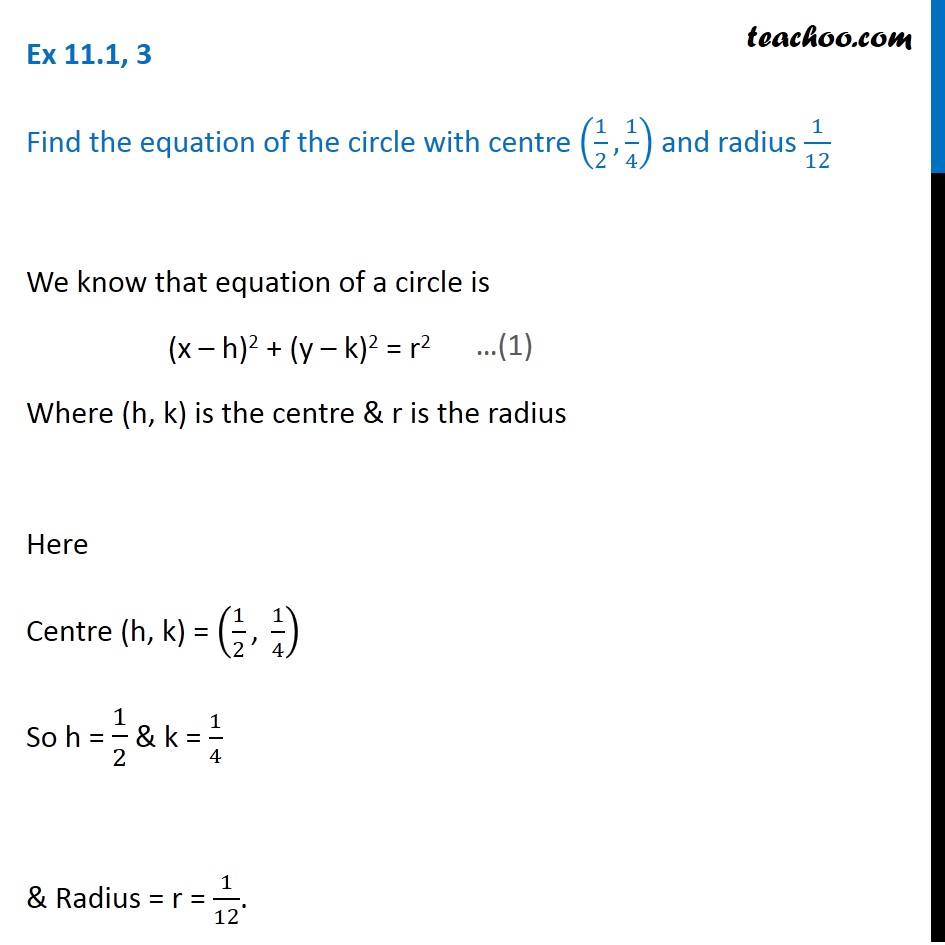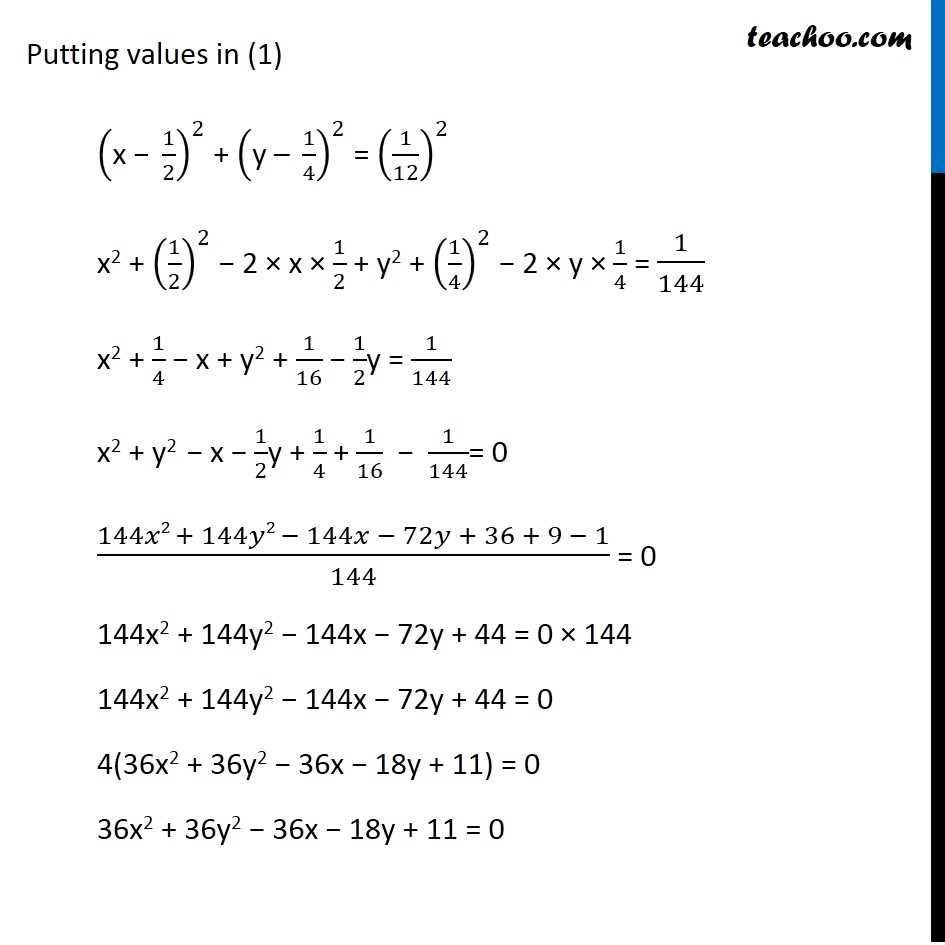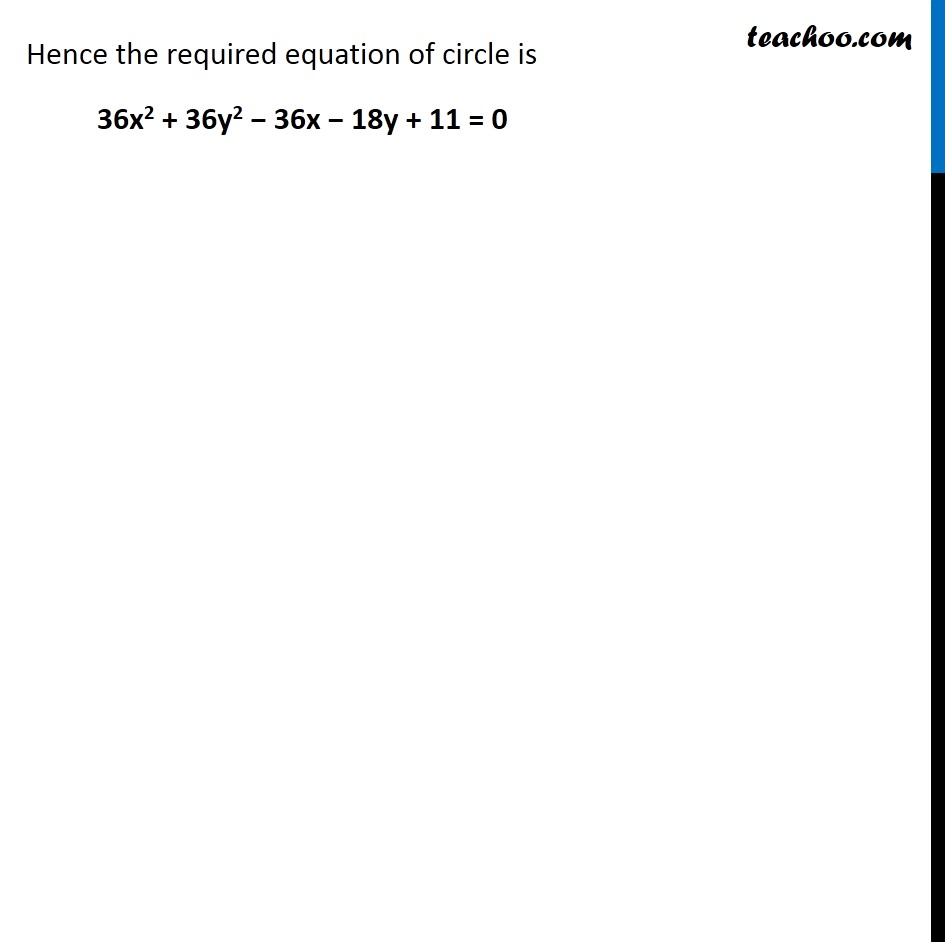Circle

Chapter 11 Class 11 Conic Sections
Concept wiseIntroducing your new favourite teacher - Teachoo Black, at only ₹83 per month

### Transcript

Ex 11.1, 3 Find the equation of the circle with centre (1/2,1/4) and radius 1/12 We know that equation of a circle is (x­ – h)2 + (y ­– k)2 = r2 Where (h, k) is the centre & r is the radius Here Centre (h, k) = (1/2 ", " 1/4) So h = 1/2 & k = 1/4 & Radius = r = 1/12. Putting values in (1) ("x − " 1/2)^2 + ("y – " 1/4)^2 = (1/12)^2 x2 + (1/2)^2 − 2 × x × 1/2 + y2 + (1/4)^2 − 2 × y × 1/4 = 1/144 x2 + 1/4 − x + y2 + 1/16 − 1/2y = 1/144 x2 + y2 − x − 1/2y + 1/4 + 1/16 − 1/144= 0 (144𝑥2 + 144𝑦2 − 144𝑥 − 72𝑦 + 36 + 9 − 1)/144 = 0 144x2 + 144y2 − 144x − 72y + 44 = 0 × 144 144x2 + 144y2 − 144x − 72y + 44 = 0 4(36x2 + 36y2 − 36x − 18y + 11) = 0 36x2 + 36y2 − 36x − 18y + 11 = 0 Hence the required equation of circle is 36x2 + 36y2 − 36x − 18y + 11 = 0